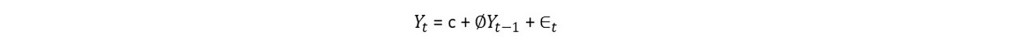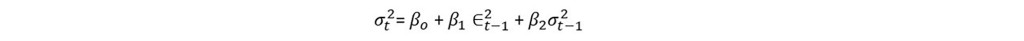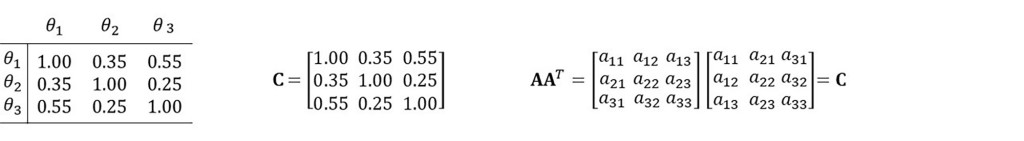A Framework for Multiple Economic Scenarios Under CECLBY ROBERT CHANG AND MARK JORDAN, FI CONSULTING
Background
We present a pragmatic approach to generating multiple economic scenarios for the new FASB current expected credit loss (CECL) accounting standard. While the guidance does not explicitly mention the number of scenarios that should be used when measuring expected credit losses, financial institutions should consider a range of economic conditions in their forecasts due to the greater accuracy and stability that multiple scenarios will provide. Evaluating a range of possible outcomes will help account for path dependencies and non-linearities that are likely present in the loss calculation. Depending on the asset and model type, the path taken by a macroeconomic variable can influence the credit outcome. Asset concentrations and correlations between economic assumptions can result in expected losses being overstated or understated if only one scenario is used.
Challenges
Institutions face data availability challenges in developing scenarios that encompass both favorable and unfavorable outcomes. Entities may have consensus “baseline” scenarios, but lack an economic research group to generate a range of outcomes. DFAST/CCAR scenarios can provide guidance, but there are important differences between the DFAST/CCAR scenarios and what is expected under CECL. DFAST/CCAR scenarios are deterministic and not probability weighted, though experts have calculated that the baseline scenario is intended to correspond to 50% probability, and the Adverse and Severely Adverse scenarios to 10% and 4% respectively.
Even when entities have multiple economic scenarios, it is difficult to assign probabilities in a way that is not judgmental. In fact, the probability of any single, specific scenario occurring is very low. It is easier to assign probabilities to scenario ranges, e.g., a scenario equal or worse will occur XX% of the time. Furthermore, economic assumptions are not perfectly correlated and do not move in lockstep. A downside scenario that applies the same amount of stress to the unemployment rate, stock market level, and home price index simultaneously will likely overstate expected losses due to imperfect correlation.
An ideal methodology for generating multiple economic scenarios will include the following:

1. Empirically driven probability weightings
2. Upside/Downside scenarios that are in part derived from historical experience
3. A joint probability distribution between economic assumptions

An Example Framework
We demonstrate a framework that empirically derives scenarios and accounts for correlation between macroeconomic assumptions. Like many modeling choices related to CECL, different approaches are possible and any methodology chosen will need to be periodically re-assessed. Our example framework is not computationally cumbersome, and uses only an upside, downside, and baseline scenario. The methodology accommodates institutions that already have baseline scenarios, but are interested in generating upside and downside scenarios that align with both their baseline scenario and historical experience.
Step 1: Model the scenario variable as an autoregressive (AR) time series with random disturbances having conditional heteroscedastic variances (e.g. GARCH).
The AR(1) process takes the following form:where ∈t is iid N (0, σ2 ), and where the variance (σ2) of the residual term follows a GARCH (1,1) process:where ∈ is the error term from the AR(1)  process.
Step 2: Use maximum likelihood estimation (MLE) to estimate the AR and GARCH parameters. The time-period used to estimate the parameters will differ depending on the portfolio type, but should, at a minimum, cover several business cycles.
Step 3: Use the model based on these parameters to simulate future values. When the simulation is implemented, the “constant” term c is calibrated to match the baseline forecast. The parameter is chosen so that the baseline forecast is the median value among 100 simulations. This approach has been used to forecast economic scenarios for lifetime loss modeling on large Federal portfolios.
Step 4: Rank the 100 simulations in order of severity with the 0th percentile scenario being the worst-case scenario and the 100th being the best-case scenario. The 10th percentile scenario is chosen as the unfavorable scenario, and is considered representative of the loss arising for all scenarios between the 0th and 25th percentiles. This scenario is assigned a 25% weighting, not a 10% weighting.
Similarly, the 90th percentile scenario is chosen to represent the gain arising for all scenarios between the 75th and 100th percentiles, and assigned a 25% weighting. The baseline forecast is assigned a 50% weighting as it represents an outcome in which there is a 50% probability that economic conditions will be worse and a 50% probability that economic conditions will be better over the duration of the forecast.
Correlation of Economic Assumptions
We then generate correlated scenarios for each of our economic assumptions. Accounting for correlation between economic assumptions will produce realistic scenarios that do not overstate or understate the overall amount of macroeconomic stress.
Step 1: Estimate AR-GARCH equations for each of our economic assumptions as described above.
Step 2: Create a variance/covariance matrix of economic assumptions. The matrix can be created from standard linear regression techniques that consider the average dependence between assumptions.
Step 3: Generate correlated normally distributed random variables to use for forecasting. First generate uncorrelated samples, then multiply them by a matrix A such that AAT=C, where C is the covariance matrix. Matrix A can be created by using the Cholesky decomposition of C, or from the eigenvalues and eigenvectors of C.Step 4: Generate the favorable and unfavorable scenarios as described previously, but instead use the correlated normally distributed random variables to produce the forecast.
Conclusion
Determining scenarios that are representative of a range of economic conditions for the purposes of measuring CECL will challenge many institutions. We have presented an accessible framework that can be easily communicated to the board, auditors, and other key stakeholders, and will not require additional costly data and modeling investments. Most importantly, our framework does not rely on judgmental probability weightings, but is centered on a baseline forecast and historical experience. Finally, correlation between economic assumptions is accounted for, resulting in realistic scenarios that do not overstate or understate expected losses.